﻿ 快速的圆投影图像匹配算法
«上一篇文章快速检索 高级检索

 智能系统学报2020, Vol. 15Issue (1): 84-91  DOI: 10.11992/tis.2019030370

### 引用本文CAO Tian, LI Bo, REN Fuji, et al. Fast image matching algorithm based on circular projection[J]. CAAI Transactions on Intelligent Systems, 2020, 15(1): 84-91. DOI: 10.11992/tis.201903037.### 文章历史

1. 南京大学 电子科学与工程学院，江苏 南京 210046;
2. 合肥工业大学 计算机与信息学院，安徽 合肥 230009;
3. 德岛大学 智能信息工学部，日本 德岛 7708500;
4. 南通大学 电子与信息学院，江苏 南通 226019

Fast image matching algorithm based on circular projection
CAO Tian 1, LI Bo 1, REN Fuji 2,3, DONG Rong 4
1. School of Electronic Science and Engineering, Nanjing University, Nanjing 210046, China;
2. School of Computer and Information Science, Hefei University of Technology, Hefei 230009, China;
3. Department of Information Science and Intelligent Systems, Tokushima University, Tokushima 7708500, Japan;
4. School of Electronics and Information, Nantong University, Nantong 226019, China
Abstract: In view of the high computational complexity and the incapability to recognize homogeneous regions of existing circular projection matching algorithms, a new image matching algorithm is proposed in this study. On the basis of hybrid circular projection and combined with pyramid decomposition and angle histogram estimation, the proposed algorithm can not only identify the exact position of the template in the image to be matched but also obtain the rotation angle of the template through the angle estimation strategy. First, candidate points are identified by the image pyramid strategy combined with the hybrid circular projection vector. Then, the positions of the candidate points are precisely located and determined. Finally, the exact angle is calculated by the angle histogram algorithm. The experimental results show the high recognition rate and fast matching speed of the proposed algorithm.
Key words: circular projection    template matching    image matching    image pyramid    angle histogram    hybrid circular projection    topmost local clustering    nonmaximal suppression

1 标准圆投影匹配算法

 $P(r) = \sum\limits_{\theta = 0}^{2\pi } {T(r,\theta )} ,\;0 \leqslant r \leqslant R$ (1)Download: 图 1 圆投影模板示意图 Fig. 1 Circular projection template

 ${ P} = (p(0),p(1), \cdots ,p(R))$ (2)

 $P(r) = \frac{1}{{n(r)}}\sum\limits_{\theta = 0}^{2\pi } {T(r,\theta )} ,\;0 \leqslant r \leqslant R$ (3)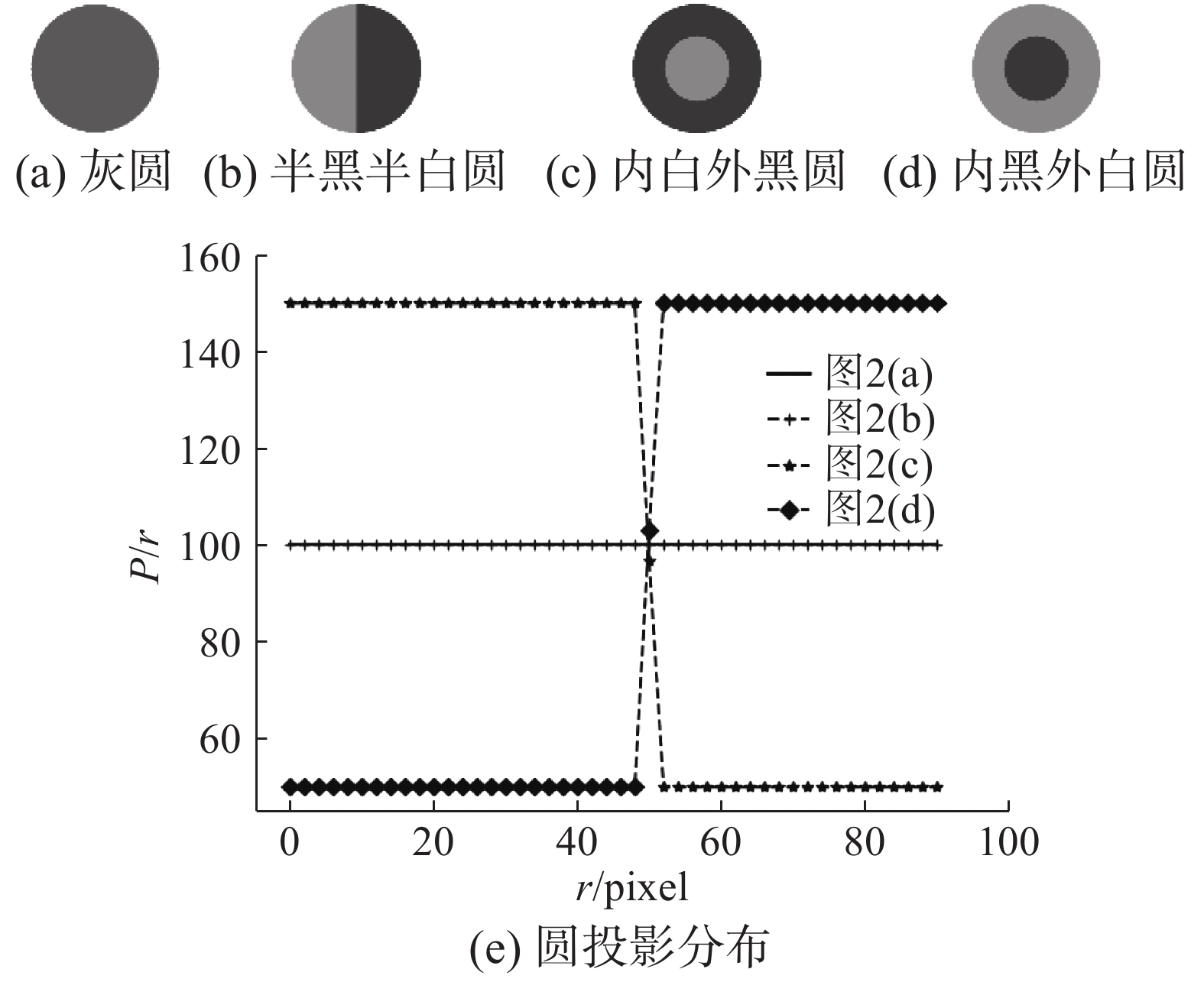Download: 图 2 简单图和对应的圆投影向量 Fig. 2 Simple graph and corresponding circular projection vector
2 改进的圆投影算法 2.1 圆投影向量的改进

 $\sigma (r) = \sqrt {\frac{1}{{n(r)}}\sum\limits_{\theta = 0}^{2\pi } {{{[T(r,\theta ) - C(r)]}^2}} }$ (4)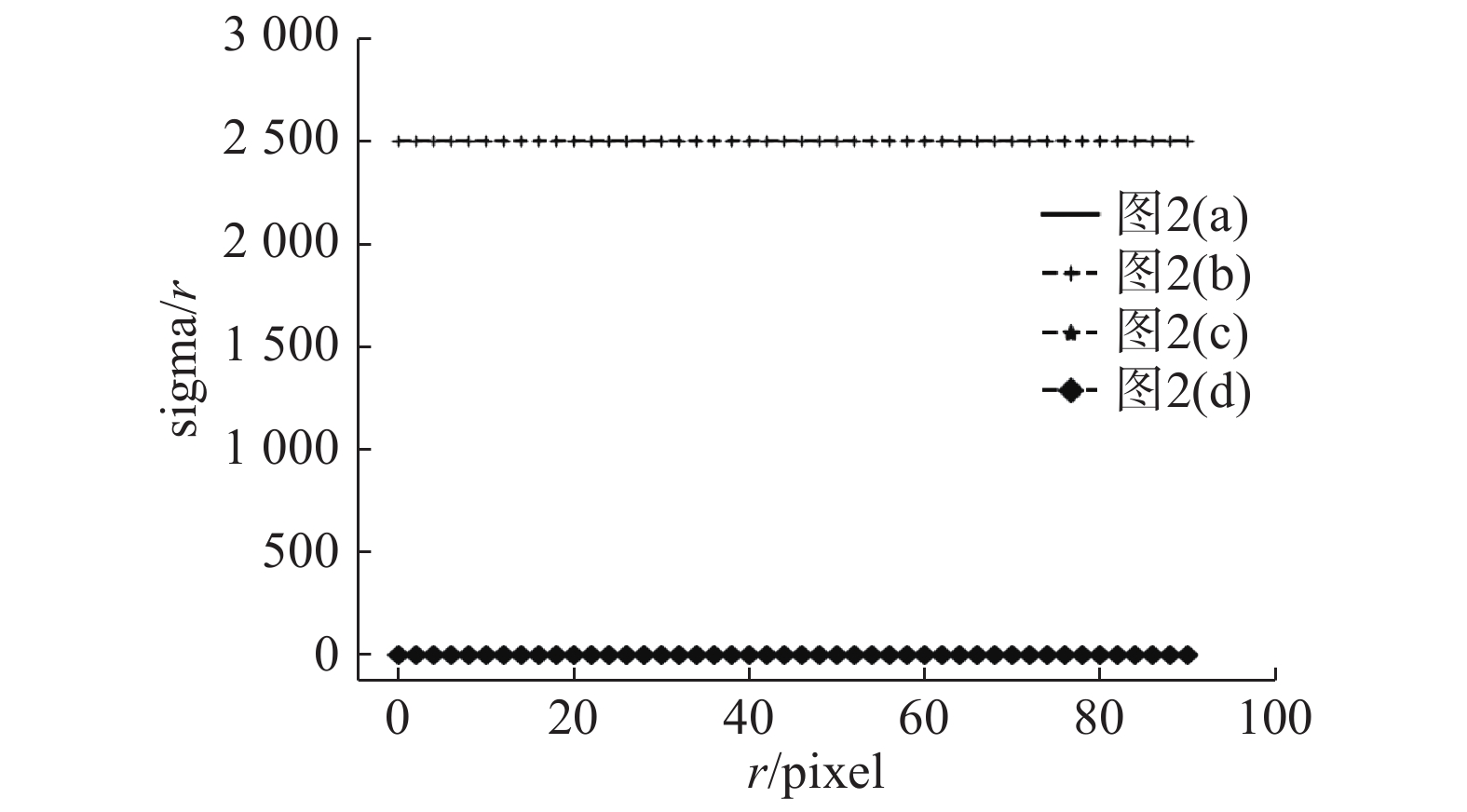Download: 图 3 方差投影向量 Fig. 3 Variance projection vector

 ${H_p}(r) = {\omega _m} \times {P_p}(r) + {\omega _\sigma } \times {\sigma _p}(r)$ (5)
 ${H_s}(r) = {\omega _m} \times {P_s}(r) + {\omega _\sigma } \times {\sigma _s}(r)$ (6)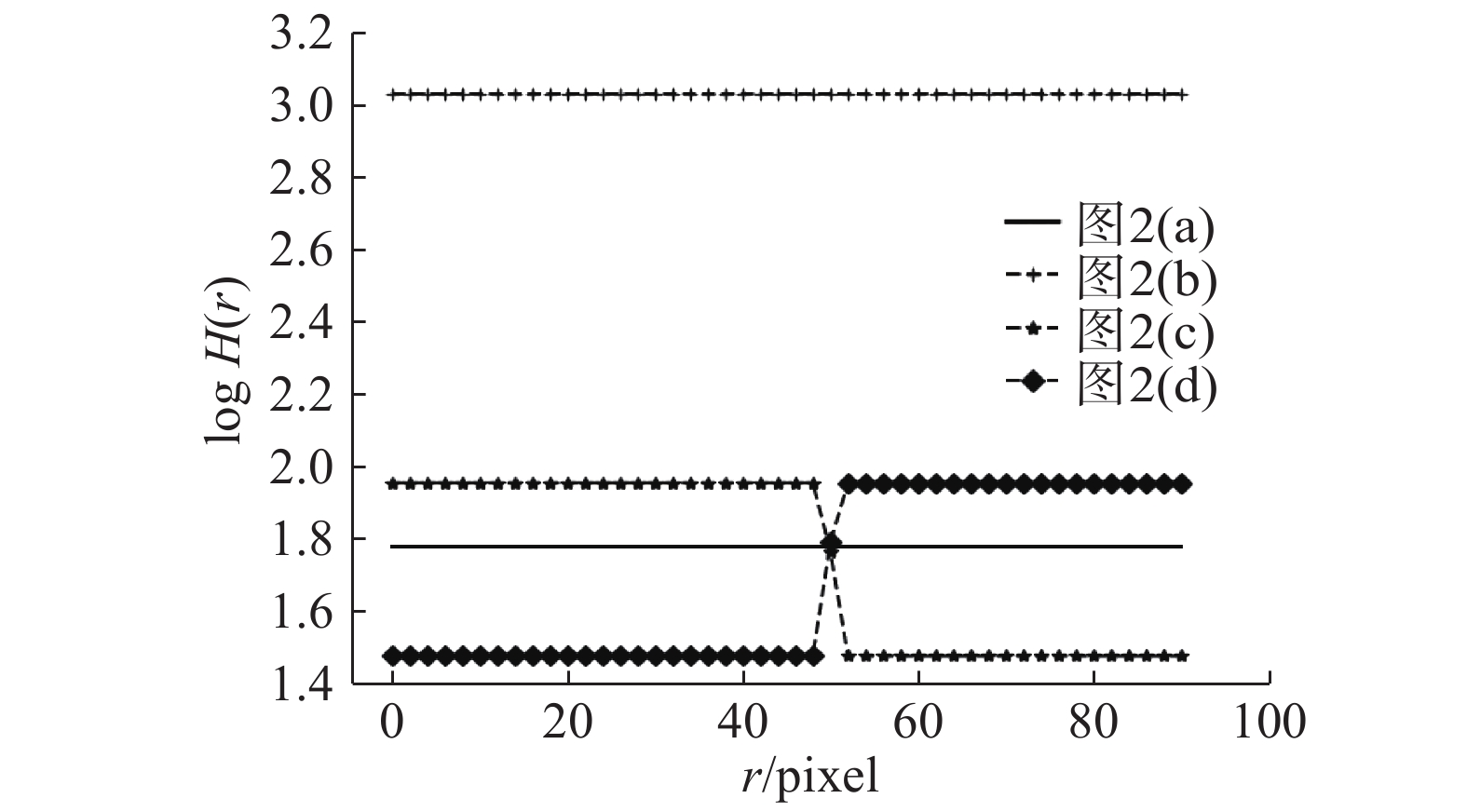Download: 图 4 混合投影向量 Fig. 4 Hybrid projection vector
2.2 相似度函数

 $f = \dfrac{{\displaystyle\sum\limits_{r = {R_{\min }}}^{{R_{\max }}} {[{H_p}(r) - \overline {{H_p}} ] \times [{H_s}(r) - \overline {{H_s}} ]} }}{{\displaystyle\sum\limits_{r = {R_{\min }}}^{{R_{\max }}} {{{[{H_p}(r) - \overline {{H_p}} ]}^2}} \times \displaystyle\sum\limits_{r = {R_{\min }}}^{{R_{\max }}} {{{[{H_s}(r) - \overline {{H_s}} ]}^2}} }}$ (7)

2.3 图像金字塔加速

2.3.1 顶层局部聚类

3) 计算下一个点，重复步骤2)，直到所有的点遍历完，计算结束。

4) 最终将hash_table中的点位置保存下来。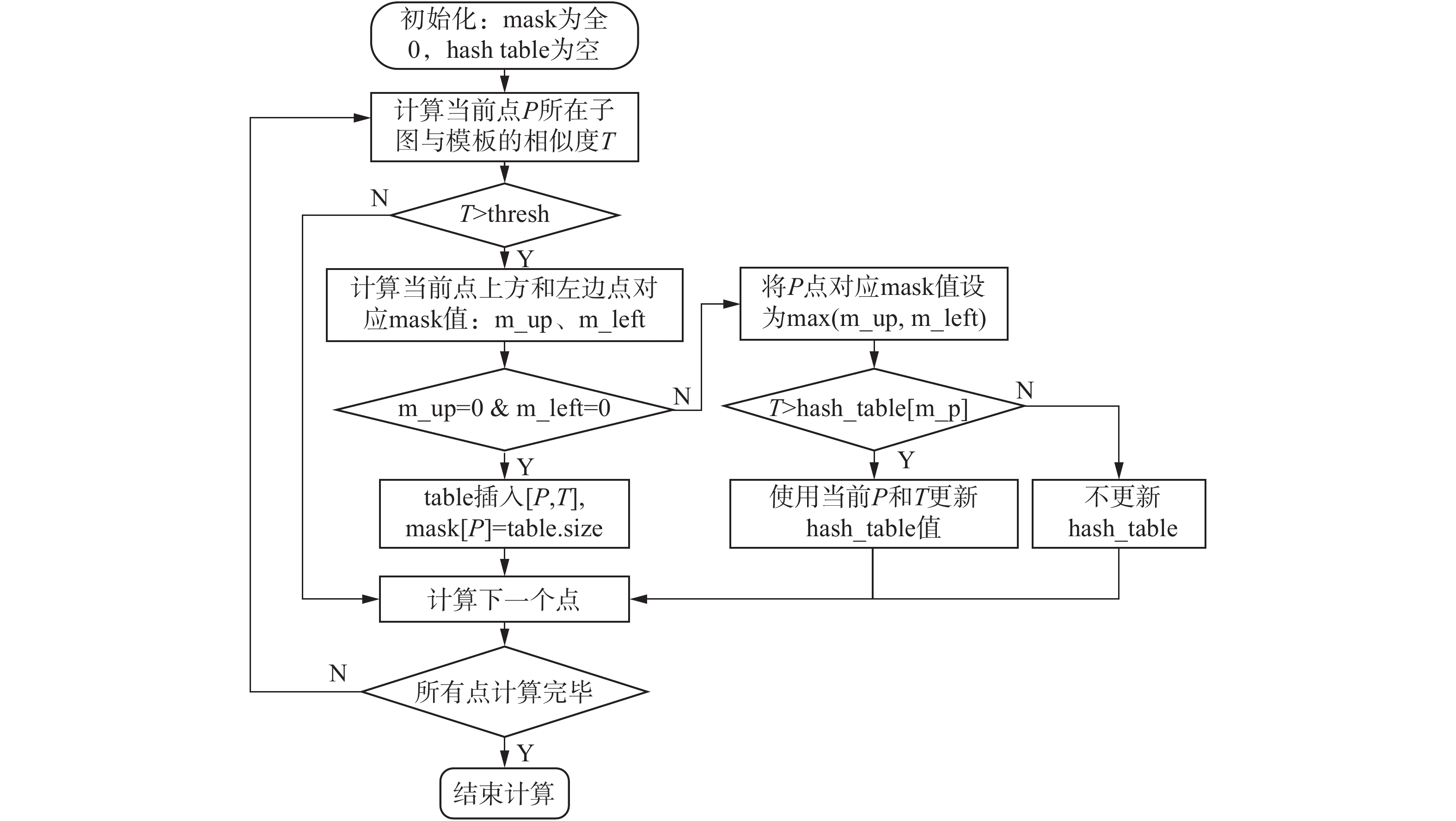Download: 图 5 顶部层次聚类流程图 Fig. 5 Top hierarchical clustering flowchart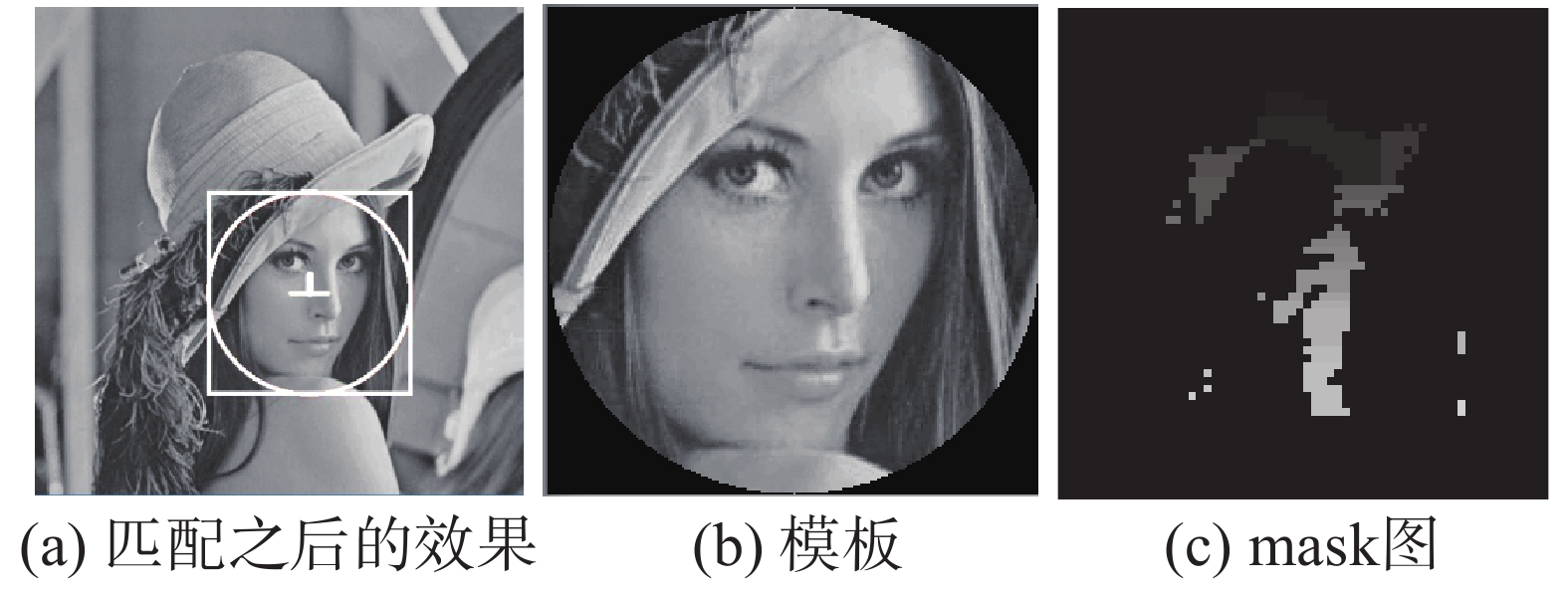Download: 图 6 局部聚类效果 Fig. 6 Sketch of local clustering

2.3.2 逐层金字塔筛选

NMS的基本思想是保留局部最大值、抑制非极大值，计算流程如下：对于候选点列表B及其对应的相似度S，结果候选点集合为D，采用下面的计算方式：

1) 将候选点集合B根据相似度得分进行降序排列。

2) 选择具有最大得分的点P，将其从B集合中移除并加入到最终的候选点集合D中。

3) 计算B中剩余候选点中与P距离dis，将dis小于阈值d(此处阈值一般选择为模板的内切圆半径) 的点从B中移除。

4) 重复这个过程，直到B为空或D中候选点数已超过一定数量。此时D中保留的候选点，作为本层最终的候选点集。

NMS流程示意图如图7所示。刚开始O1~O4计算得到的分值分别为0.9、0.7、0.6、0.8；通过选择最大得分O1加入候选集D并在B中删除，删除B中与O1距离在r以内的点(O2O3)，在B中选择最大得分的点(仅有O4)，加入到D中，并将其从B中删除，得到D{O1O4}。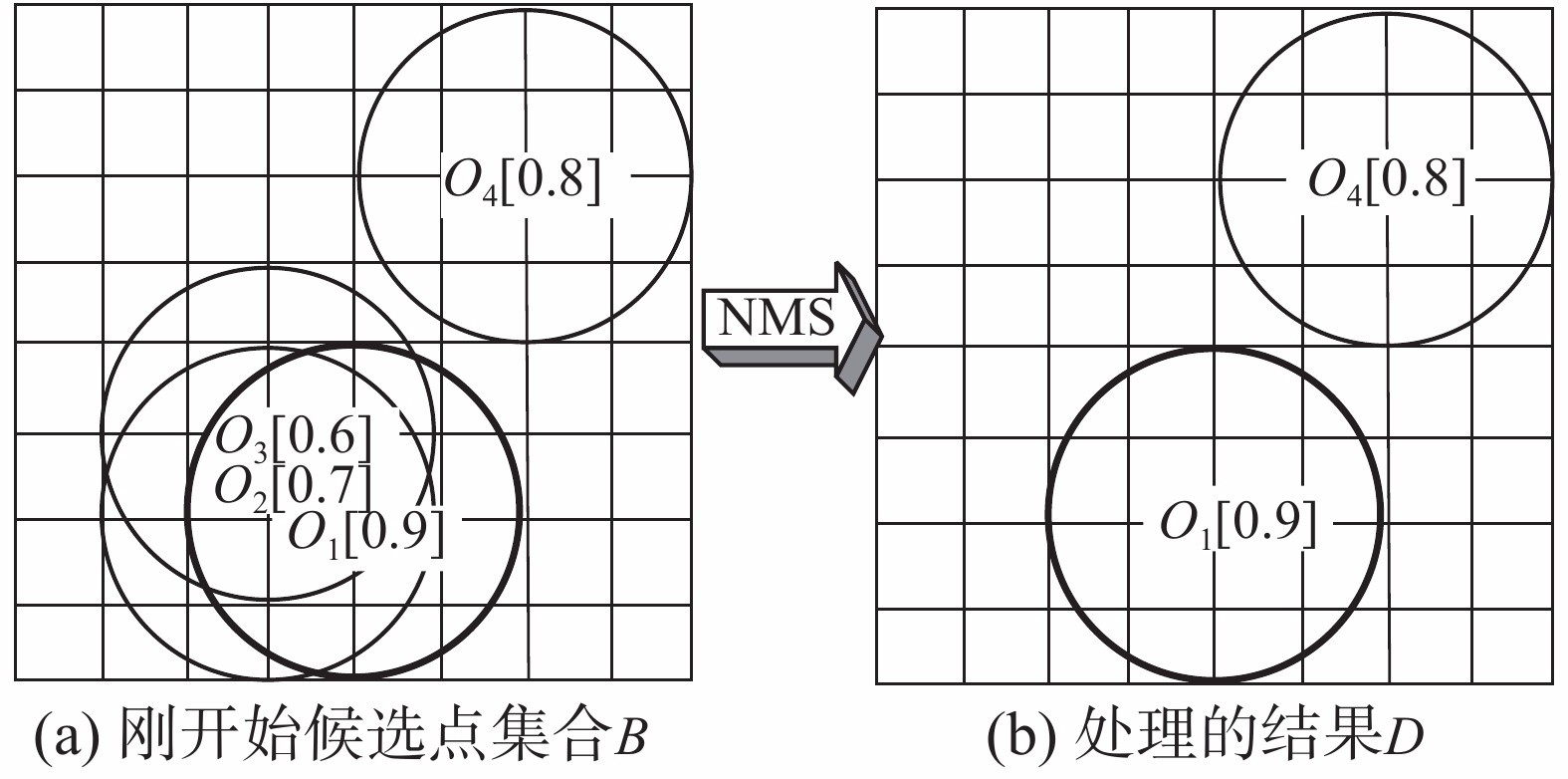Download: 图 7 非极大值抑制示意图 Fig. 7 Diagram of NMS

2.4 角度估计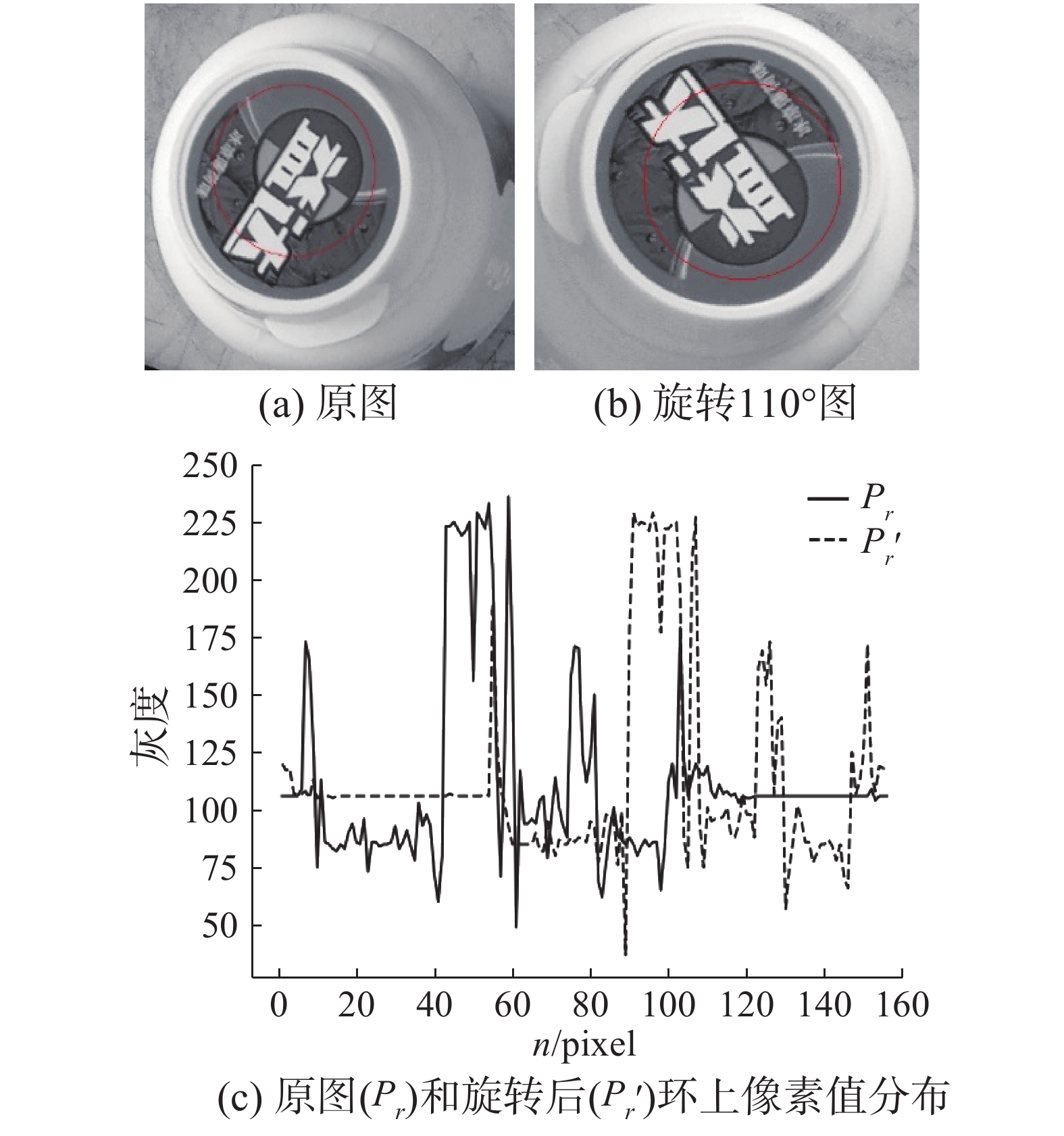Download: 图 8 环上像素值分布 Fig. 8 Pixel value distributions in rings

 ${\theta _r} = {k_r} \times {\varphi _r}$ (8)

 ${k_r} = \arg \max ({\delta _r}(k)),k \in [0,{N_r}]$ (9)

${N_r}$ 为半径为r的环上像素数量。 ${\delta _r}(k)$ 为偏移k像素之后和模板中环的归一化互相关系数，定义为

 ${\delta _r}(k) = \frac{{\displaystyle\sum\limits_{n = 0}^{{N_r}} {[{{{p}}_r}(n) - \overline {{{{p}}_r}(n)} ] \times [{{{p}}_r}'(n) - \overline {{{{p}}_r}'(n)} ]} }}{{\displaystyle\sum\limits_{n = 0}^{{N_r}} {{{[{{{p}}_r}(n) - \overline {{{{p}}_r}(n)} ]}^2}} \times\sum\limits_{n = 0}^{{N_r}} {{{[{{{p}}_r}'(n) - \overline {{{{p}}_r}'(n)} ]}^2}} }}$ (10)

1)构建一个维数为360的数组a，存放旋转角度对应的环的数量。

2)计算半径为r处的旋转角度 ${\theta _r}$ ，四舍五入后angle放入直方图，即a[angle]加1。

3)对所有半径进行步骤2)，最终构建角度分布的直方图。

4)检查步骤3)得到的角度直方图中的众数，即为最终角度。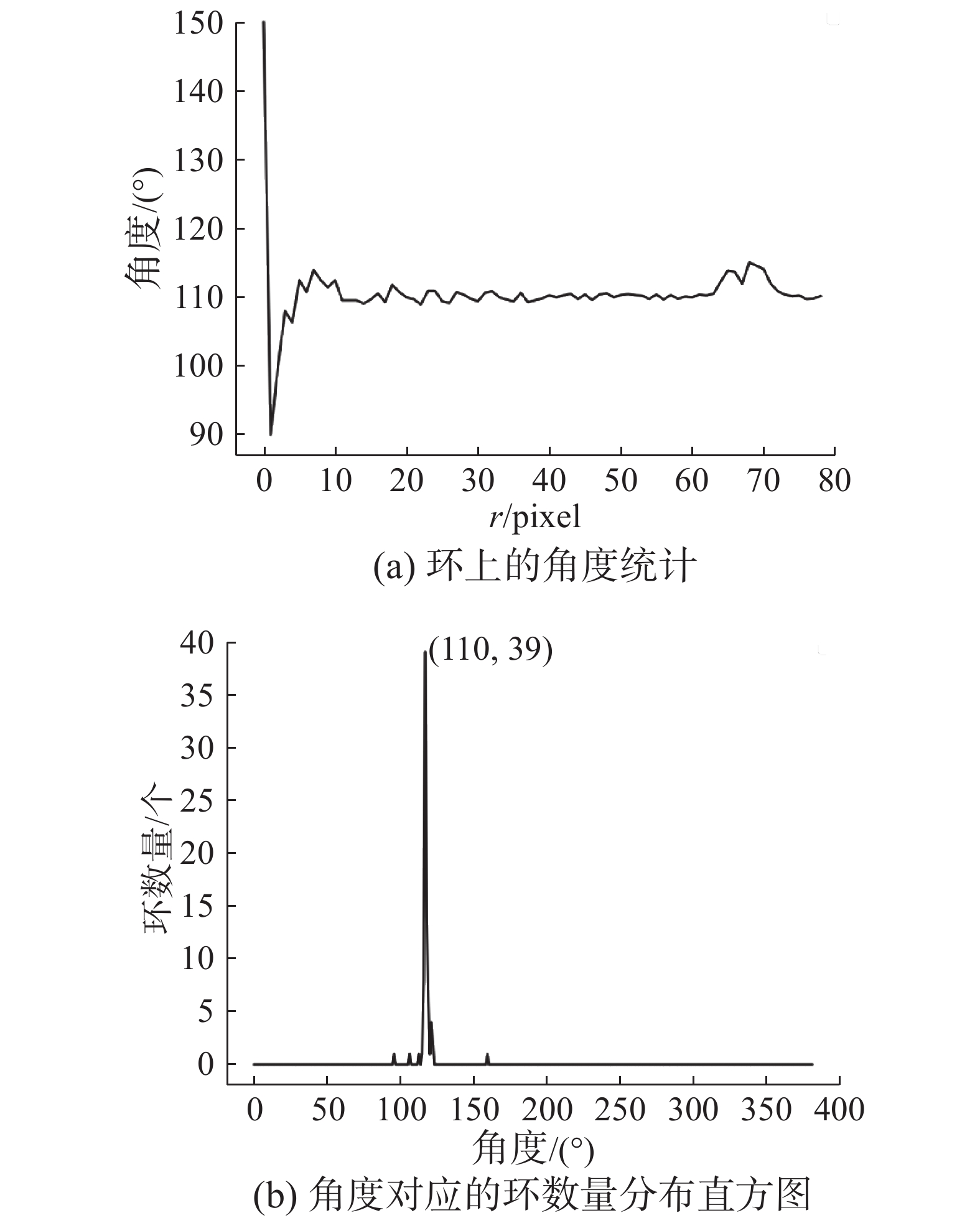Download: 图 9 环上角度统计及分布直方图 Fig. 9 Angle statistics and distribution histogram

2.5 计算复杂度分析

3 实验结果

3.1 光照变化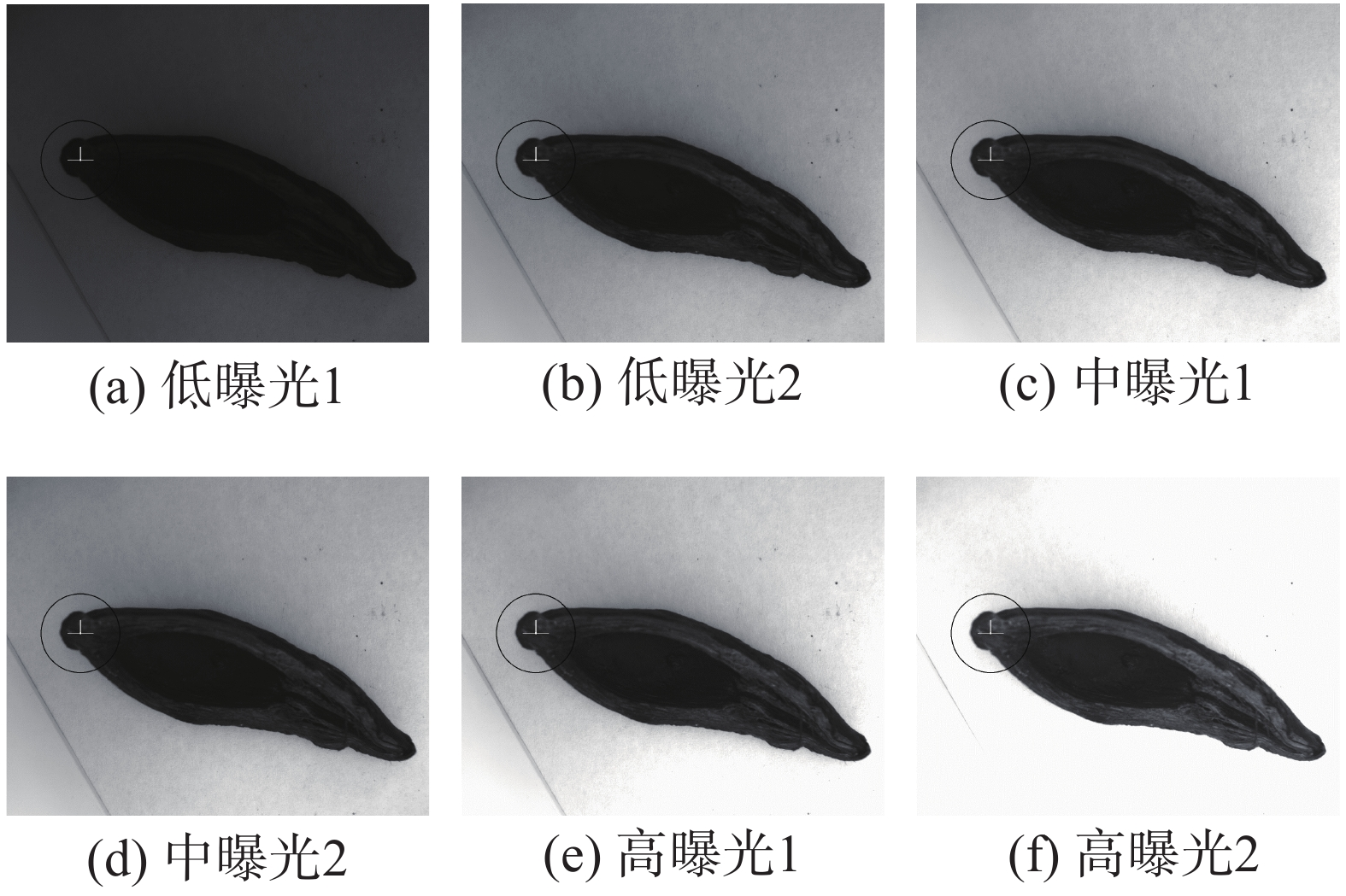Download: 图 10 不同光照下匹配情况 Fig. 10 Matching results under different illuminations
3.2 角度估计结果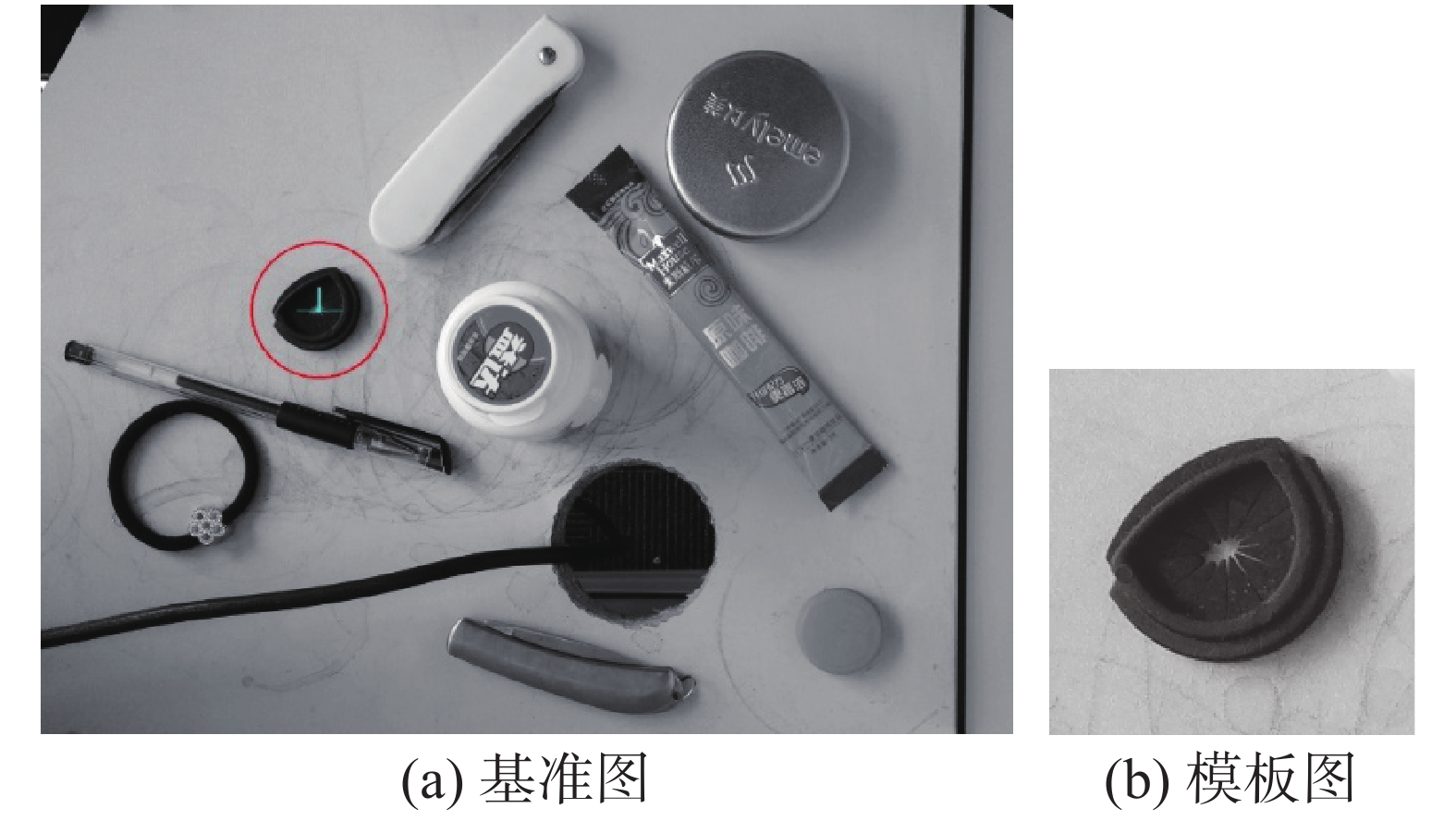Download: 图 11 旋转测试图片 Fig. 11 Rotation test pictures表 1 旋转角度匹配结果 Tab.1 Matching results for rotation test

3.3 运行速度测试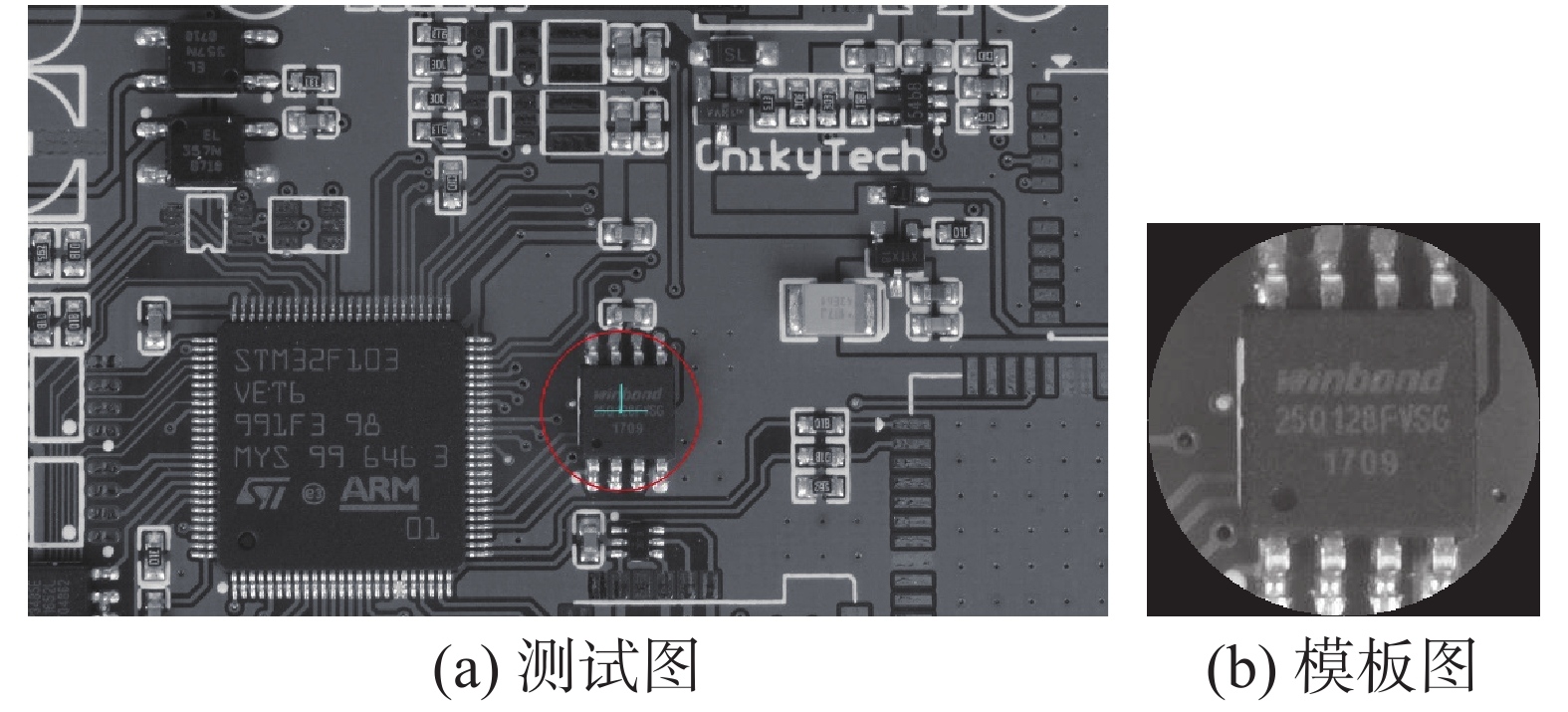Download: 图 12 性能测试图片 Fig. 12 Efficiency test pictures表 2 运行时间 Tab.2 Running time

4 结束语

  周可, 秦世引. SIFT特征匹配的辐射畸变图像相对校正新方法[J]. 智能系统学报, 2011, 6(6): 507-514. ZHOU Ke, QIN Shiyin. A novel method for relative correction of a radiometric distortion image based on SIFT feature matching[J]. CAAI transactions on intelligent systems, 2011, 6(6): 507-514. DOI:10.3969/j.issn.1673-4785.2011.06.005 (0)  阮晓虎, 李卫军, 覃鸿, 等. 一种基于特征匹配的人脸配准判断方法[J]. 智能系统学报, 2015, 10(1): 12-19. RUAN Xiaohu, LI Weijun, QIN Hong, et al. An assessment method for face alignment based on feature matching[J]. CAAI transactions on intelligent systems, 2015, 10(1): 12-19. (0)  李龙, 尹辉, 许宏丽, 等. 一种鲁棒的Multi-Egocentric视频中的多目标检测及匹配算法[J]. 智能系统学报, 2016, 11(5): 619-626. LI Long, YIN Hui, XU Hongli, et al. A robust multi-object detection and matching algorithm for multi-egocentric videos[J]. CAAI transactions on intelligent systems, 2016, 11(5): 619-626. (0)  王杰, 蒋明敏, 花晓慧, 等. 基于投影直方图匹配的双目视觉跟踪算法[J]. 智能系统学报, 2015, 10(5): 775-782. WANG Jie, JIANG Mingmin, HUA Xiaohui, et al. Binocular object tracking method using projection histogram matching[J]. CAAI transactions on intelligent systems, 2015, 10(5): 775-782. (0)  ZITOVÁ B, FLUSSER J. Image registration methods: a survey[J]. Image and vision computing, 2003, 21(11): 977-1000. DOI:10.1016/S0262-8856(03)00137-9 (0)  AGGARWAL J K, DAVIS L S, MARTIN W N. Correspondence processes in dynamic scene analysis[J]. Proceedings of the IEEE, 1981, 69(5): 562-572. DOI:10.1109/PROC.1981.12025 (0)  SECILLA J P, GRACIA N, CARRASCOSA J L. Template location in noisy pictures[J]. Signal processing, 1988, 14(4): 347-361. DOI:10.1016/0165-1684(88)90093-X (0)  TANIMOTO S L. Template matching in pyramids[J]. Computer graphics and image processing, 1981, 16(4): 356-369. DOI:10.1016/0146-664X(81)90046-0 (0)  ROSENFELD A, KAK A, Digital image processing[M]. 2nd ed. Orlando: Academic Press, 1982. (0)  LOWE D G. Distinctive image features from scale-invariant keypoints[J]. International journal of computer vision, 2004, 60(2): 91-110. DOI:10.1023/B:VISI.0000029664.99615.94 (0)  郑永斌, 黄新生, 丰松江. SIFT和旋转不变LBP相结合的图像匹配算法[J]. 计算机辅助设计与图形学学报, 2010, 22(2): 286-292. ZHENG Yongbin, HUANG Xinsheng, FENG Songjiang. An image matching algorithm based on combination of SIFT and the rotation invariant LBP[J]. Journal of computer-aided design & computer graphics, 2010, 22(2): 286-292. (0)  TANG Y Y, CHENG H D, SUEN C Y. Transformation-ring-projection (TRP) algorithm and its VLSI implementation[J]. International journal of pattern recognition and artificial intelligence, 1991, 5(1/2): 25-56. (0)  TSAI D M, TSAI Y H. Rotation-invariant pattern matching with color ring-projection[J]. Pattern recognition, 2002, 35(1): 131-141. DOI:10.1016/S0031-3203(00)00180-1 (0)  LEE W C, CHEN C H. A fast template matching method with rotation invariance by combining the circular projection transform process and bounded partial correlation[J]. IEEE signal processing letters, 2012, 19(11): 737-740. DOI:10.1109/LSP.2012.2212010 (0)  徐亦斌, 王敬东, 李鹏. 基于圆投影向量的景象匹配方法研究[J]. 系统工程与电子技术, 2005, 27(10): 1725-1728. XU Yibin, WANG Jingdong, LI Peng. Research on scene matching method using circular projection[J]. Systems engineering and electronics, 2005, 27(10): 1725-1728. DOI:10.3321/j.issn:1001-506X.2005.10.017 (0)  CHOI M S, KIM W Y. A novel two stage template matching method for rotation and illumination invariance[J]. Pattern recognition, 2002, 35(1): 119-129. DOI:10.1016/S0031-3203(01)00025-5 (0)  贾晓芬, 赵佰亭, 周孟然, 等. 采用圆投影和序贯相似检测的图像匹配技术[J]. 哈尔滨商业大学学报(自然科学版), 2015, 31(2): 232-236, 241. JIA Xiafen, ZHAO Baiting, ZHOU Mengran, et al. Fast image matching algorithm based on circular projection and sequential similarity detection[J]. Journal of Harbin University of Commerce (Natural Sciences Edition), 2015, 31(2): 232-236, 241. DOI:10.3969/j.issn.1672-0946.2015.02.026 (0)  于辉, 张忠秋, 何周灿. 用于任意旋转角度景象匹配的圆投影算法[J]. 计算机工程与应用, 2011, 47(5): 172-174. YU Hui, ZHANG Zhongqiu, HE Zhoucan. Ring projection transformation algorithm for arbitrary rotation matching[J]. Computer engineering and applications, 2011, 47(5): 172-174. DOI:10.3778/j.issn.1002-8331.2011.05.052 (0)  SASSANAPITAK S, KAEWTRAKULPONG P. An efficient translation-rotation template matching using pre-computed scores of rotated templates[C]//Proceedings of the 6th International Conference on Electrical Engineering/Electronics, Computer, Telecommunications and Information Technology. Pattaya, Chonburi, Thailand: IEEE, 2009: 1040–1043. (0)